# Principal Component Analysis (PCA) From Scratch

1. Normalizing the Data
`#Importing necessary modulesimport numpy as npimport matplotlib.pyplot as pltx1_norm=x1-x1.mean()#X1' normalized from X1x2_norm=x2-x2.mean()#X2' normalized from X2`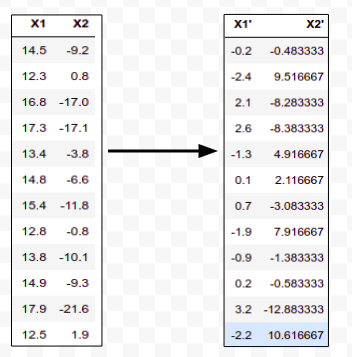Raw Data Converted into Zero mean Normalized Data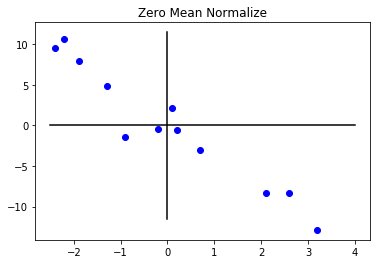Visualization of Raw data and Zero mean normalized data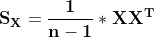Covariance matrix where X is a column matrix of dimension (m*1)
`X_matrix=np.array((x1_norm,x2_norm))Sx=(1/(X_matrix.shape-1))*np.matmul(X_matrix,np.transpose(X_matrix))OutputSx=array([[  3.51818182, -13.52454545],       [-13.52454545,  55.58878788]])(Since non-diagonal element of the matrix Sx are negative. So, we asssume both X1 and X2 variable decrease together.)`
`# eigen_value calculationm=(Sx+Sx)/2p=(Sx*Sx-(Sx*Sx))lambda1=m+(m**2-p)**(1/2)lambda2=m-(m**2-p)**(1/2)Outputlambda1=58.89203173874124lambda2= 0.21493795822846806`
`#eigen vector calculationV1=np.array((Sx/(lambda1-Sx),1))V2=np.array((Sx/(lambda2-Sx),1))Outputeigen_vectorV1=[-0.24424066  1.        ]eigen_vectorV2=[ 4.09432244  1.        ]`
`k = lambda1 / (lambda1 + lambda2)print(str(round(k*100,2))+'% variance is explained by v1')print(str(round((1-k)*100,2))+'% variance is explained by v2')Output99.64% variance is explained by v10.36% variance is explained by v2`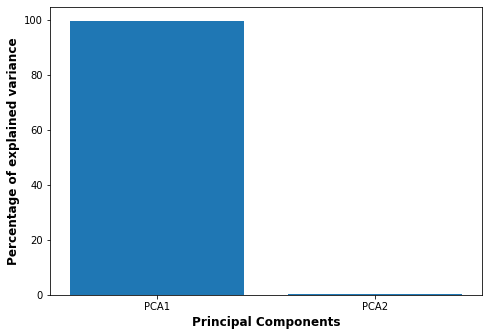The variance explained by two PCA
`P=np.array((V1,V2))Y=np.matmul(P,X_matrix)`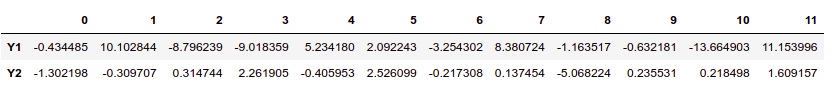Y1 and Y2 two principal component derived V1 and V2 eigenvectors respectively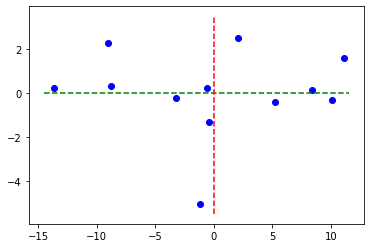Visualization of Choosing both components and finding the value of Y (No PCA)
`P=np.array((V1))#orthonormal vectorY=np.matmul(P,X_matrix)Sy=(1/(Y.shape-1))*np.matmul(Y,np.transpose(Y))OutputSy=62.40514745166816`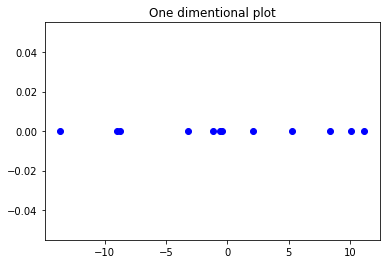One dimensional data plot with 99% of variance explained.

--

--